# Chapter 9 Clustering

## 9.1 Overview

As part of exploratory data analysis, it is often helpful to see if there are meaningful subgroups (or clusters) in the data. This grouping can be used for many purposes, such as generating new questions or improving predictive analyses. This chapter provides an introduction to clustering using the K-means algorithm, including techniques to choose the number of clusters.

## 9.2 Chapter learning objectives

By the end of the chapter, readers will be able to do the following:

• Describe a situation in which clustering is an appropriate technique to use, and what insight it might extract from the data.
• Explain the K-means clustering algorithm.
• Interpret the output of a K-means analysis.
• Differentiate between clustering and classification.
• Identify when it is necessary to scale variables before clustering, and do this using R.
• Perform K-means clustering in R using kmeans.
• Use the elbow method to choose the number of clusters for K-means.
• Visualize the output of K-means clustering in R using colored scatter plots.
• Describe the advantages, limitations and assumptions of the K-means clustering algorithm.

## 9.3 Clustering

Clustering is a data analysis technique involving separating a data set into subgroups of related data. For example, we might use clustering to separate a data set of documents into groups that correspond to topics, a data set of human genetic information into groups that correspond to ancestral subpopulations, or a data set of online customers into groups that correspond to purchasing behaviors. Once the data are separated, we can, for example, use the subgroups to generate new questions about the data and follow up with a predictive modeling exercise. In this course, clustering will be used only for exploratory analysis, i.e., uncovering patterns in the data.

Note that clustering is a fundamentally different kind of task than classification or regression. In particular, both classification and regression are supervised tasks where there is a response variable (a category label or value), and we have examples of past data with labels/values that help us predict those of future data. By contrast, clustering is an unsupervised task, as we are trying to understand and examine the structure of data without any response variable labels or values to help us. This approach has both advantages and disadvantages. Clustering requires no additional annotation or input on the data. For example, while it would be nearly impossible to annotate all the articles on Wikipedia with human-made topic labels, we can cluster the articles without this information to find groupings corresponding to topics automatically. However, given that there is no response variable, it is not as easy to evaluate the “quality” of a clustering. With classification, we can use a test data set to assess prediction performance. In clustering, there is not a single good choice for evaluation. In this book, we will use visualization to ascertain the quality of a clustering, and leave rigorous evaluation for more advanced courses.

As in the case of classification, there are many possible methods that we could use to cluster our observations to look for subgroups. In this book, we will focus on the widely used K-means algorithm . In your future studies, you might encounter hierarchical clustering, principal component analysis, multidimensional scaling, and more; see the additional resources section at the end of this chapter for where to begin learning more about these other methods.

Note: There are also so-called semisupervised tasks, where only some of the data come with response variable labels/values, but the vast majority don’t. The goal is to try to uncover underlying structure in the data that allows one to guess the missing labels. This sort of task is beneficial, for example, when one has an unlabeled data set that is too large to manually label, but one is willing to provide a few informative example labels as a “seed” to guess the labels for all the data.

An illustrative example

Here we will present an illustrative example using a data set from the palmerpenguins R package . This data set was collected by Dr. Kristen Gorman and the Palmer Station, Antarctica Long Term Ecological Research Site, and includes measurements for adult penguins found near there . We have modified the data set for use in this chapter. Here we will focus on using two variables—penguin bill and flipper length, both in millimeters—to determine whether there are distinct types of penguins in our data. Understanding this might help us with species discovery and classification in a data-driven way.Figure 9.1: Gentoo penguin.

To learn about K-means clustering we will work with penguin_data in this chapter. penguin_data is a subset of 18 observations of the original data, which has already been standardized (remember from Chapter 5 that scaling is part of the standardization process). We will discuss scaling for K-means in more detail later in this chapter.

Before we get started, we will load the tidyverse metapackage as well as set a random seed. This will ensure we have access to the functions we need and that our analysis will be reproducible. As we will learn in more detail later in the chapter, setting the seed here is important because the K-means clustering algorithm uses random numbers.

library(tidyverse)
set.seed(1)

Now we can load and preview the data.

penguin_data <- read_csv("data/penguins_standardized.csv")
penguin_data
## # A tibble: 18 × 2
##    flipper_length_standardized bill_length_standardized
##                          <dbl>                    <dbl>
##  1                      -0.190                   -0.641
##  2                      -1.33                    -1.14
##  3                      -0.922                   -1.52
##  4                      -0.922                   -1.11
##  5                      -1.41                    -0.847
##  6                      -0.678                   -0.641
##  7                      -0.271                   -1.24
##  8                      -0.434                   -0.902
##  9                       1.19                     0.720
## 10                       1.36                     0.646
## 11                       1.36                     0.963
## 12                       1.76                     0.440
## 13                       1.11                     1.21
## 14                       0.786                    0.123
## 15                      -0.271                    0.627
## 16                      -0.271                    0.757
## 17                      -0.108                    1.78
## 18                      -0.759                    0.776

Next, we can create a scatter plot using this data set to see if we can detect subtypes or groups in our data set.

ggplot(data, aes(x = flipper_length_standardized,
y = bill_length_standardized)) +
geom_point() +
xlab("Flipper Length (standardized)") +
ylab("Bill Length (standardized)") +
theme(text = element_text(size = 12))Figure 9.2: Scatter plot of standardized bill length versus standardized flipper length.

Based on the visualization in Figure 9.2, we might suspect there are a few subtypes of penguins within our data set. We can see roughly 3 groups of observations in Figure 9.2, including:

1. a small flipper and bill length group,
2. a small flipper length, but large bill length group, and
3. a large flipper and bill length group.

Data visualization is a great tool to give us a rough sense of such patterns when we have a small number of variables. But if we are to group data—and select the number of groups—as part of a reproducible analysis, we need something a bit more automated. Additionally, finding groups via visualization becomes more difficult as we increase the number of variables we consider when clustering. The way to rigorously separate the data into groups is to use a clustering algorithm. In this chapter, we will focus on the K-means algorithm, a widely used and often very effective clustering method, combined with the elbow method for selecting the number of clusters. This procedure will separate the data into groups; Figure 9.3 shows these groups denoted by colored scatter points.Figure 9.3: Scatter plot of standardized bill length versus standardized flipper length with colored groups.

What are the labels for these groups? Unfortunately, we don’t have any. K-means, like almost all clustering algorithms, just outputs meaningless “cluster labels” that are typically whole numbers: 1, 2, 3, etc. But in a simple case like this, where we can easily visualize the clusters on a scatter plot, we can give human-made labels to the groups using their positions on the plot:

• small flipper length and small bill length (orange cluster),
• small flipper length and large bill length (blue cluster).
• and large flipper length and large bill length (yellow cluster).

Once we have made these determinations, we can use them to inform our species classifications or ask further questions about our data. For example, we might be interested in understanding the relationship between flipper length and bill length, and that relationship may differ depending on the type of penguin we have.

## 9.4 K-means

### 9.4.1 Measuring cluster quality

The K-means algorithm is a procedure that groups data into K clusters. It starts with an initial clustering of the data, and then iteratively improves it by making adjustments to the assignment of data to clusters until it cannot improve any further. But how do we measure the “quality” of a clustering, and what does it mean to improve it? In K-means clustering, we measure the quality of a cluster by its within-cluster sum-of-squared-distances (WSSD). Computing this involves two steps. First, we find the cluster centers by computing the mean of each variable over data points in the cluster. For example, suppose we have a cluster containing four observations, and we are using two variables, $$x$$ and $$y$$, to cluster the data. Then we would compute the coordinates, $$\mu_x$$ and $$\mu_y$$, of the cluster center via

$\mu_x = \frac{1}{4}(x_1+x_2+x_3+x_4) \quad \mu_y = \frac{1}{4}(y_1+y_2+y_3+y_4).$

In the first cluster from the example, there are 4 data points. These are shown with their cluster center (flipper_length_standardized = -0.35 and bill_length_standardized = 0.99) highlighted in Figure 9.4.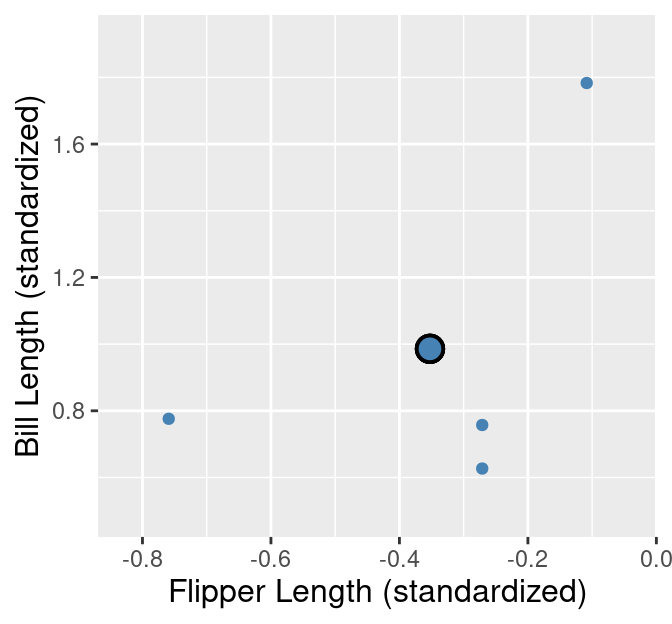Figure 9.4: Cluster 1 from the penguin_data data set example. Observations are in blue, with the cluster center highlighted in red.

The second step in computing the WSSD is to add up the squared distance between each point in the cluster and the cluster center. We use the straight-line / Euclidean distance formula that we learned about in Chapter 5. In the 4-observation cluster example above, we would compute the WSSD $$S^2$$ via

\begin{align*} S^2 = \left((x_1 - \mu_x)^2 + (y_1 - \mu_y)^2\right) + \left((x_2 - \mu_x)^2 + (y_2 - \mu_y)^2\right) + \\ \left((x_3 - \mu_x)^2 + (y_3 - \mu_y)^2\right) + \left((x_4 - \mu_x)^2 + (y_4 - \mu_y)^2\right). \end{align*}

These distances are denoted by lines in Figure 9.5 for the first cluster of the penguin data example.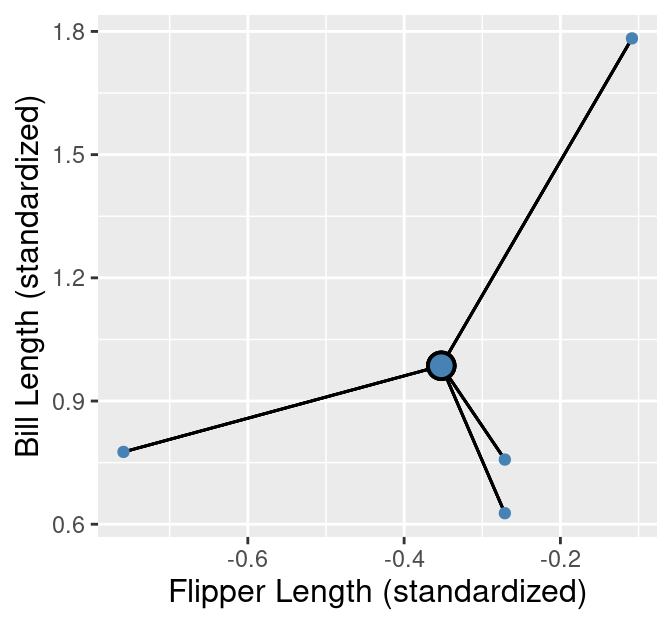Figure 9.5: Cluster 1 from the penguin_data data set example. Observations are in blue, with the cluster center highlighted in red. The distances from the observations to the cluster center are represented as black lines.

The larger the value of $$S^2$$, the more spread out the cluster is, since large $$S^2$$ means that points are far from the cluster center. Note, however, that “large” is relative to both the scale of the variables for clustering and the number of points in the cluster. A cluster where points are very close to the center might still have a large $$S^2$$ if there are many data points in the cluster.

After we have calculated the WSSD for all the clusters, we sum them together to get the total WSSD. For our example, this means adding up all the squared distances for the 18 observations. These distances are denoted by black lines in Figure 9.6.Figure 9.6: All clusters from the penguin_data data set example. Observations are in orange, blue, and yellow with the cluster center highlighted in red. The distances from the observations to each of the respective cluster centers are represented as black lines.

### 9.4.2 The clustering algorithm

We begin the K-means algorithm by picking K, and randomly assigning a roughly equal number of observations to each of the K clusters. An example random initialization is shown in Figure 9.7.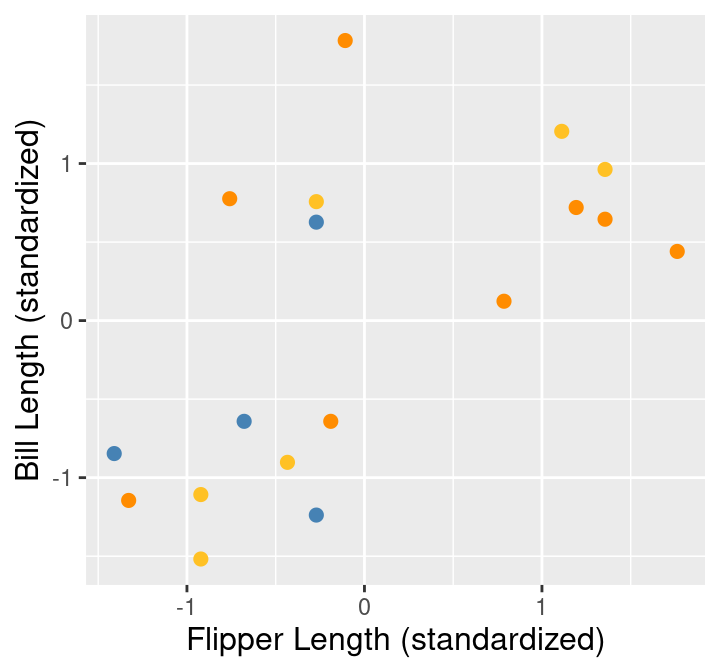Figure 9.7: Random initialization of labels.

Then K-means consists of two major steps that attempt to minimize the sum of WSSDs over all the clusters, i.e., the total WSSD:

1. Center update: Compute the center of each cluster.
2. Label update: Reassign each data point to the cluster with the nearest center.

These two steps are repeated until the cluster assignments no longer change. We show what the first four iterations of K-means would look like in
Figure 9.8. There each row corresponds to an iteration, where the left column depicts the center update, and the right column depicts the reassignment of data to clusters.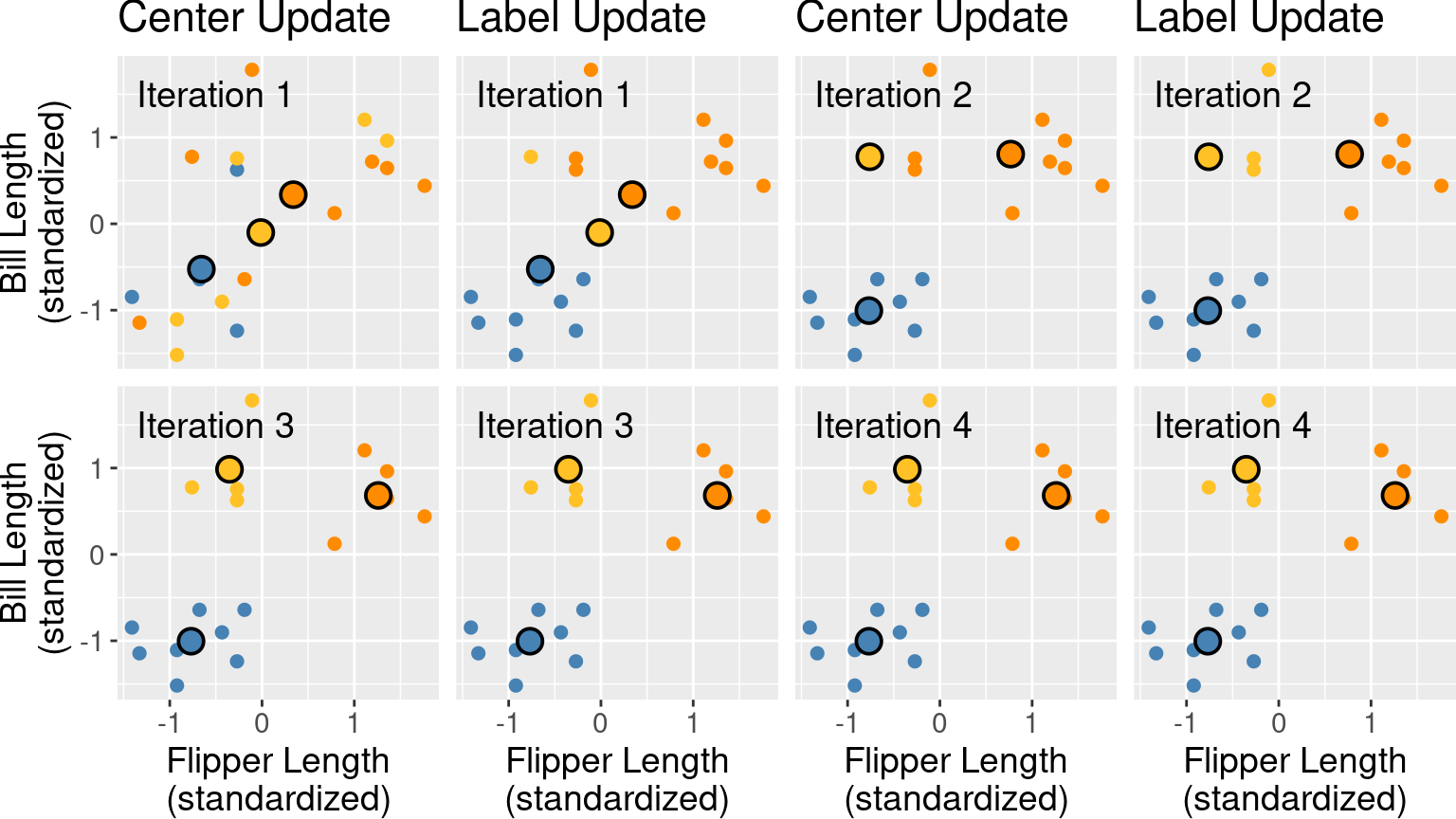Figure 9.8: First four iterations of K-means clustering on the penguin_data example data set. Each pair of plots corresponds to an iteration. Within the pair, the first plot depicts the center update, and the second plot depicts the reassignment of data to clusters. Cluster centers are indicated by larger points that are outlined in black.

Note that at this point, we can terminate the algorithm since none of the assignments changed in the fourth iteration; both the centers and labels will remain the same from this point onward.

Note: Is K-means guaranteed to stop at some point, or could it iterate forever? As it turns out, thankfully, the answer is that K-means is guaranteed to stop after some number of iterations. For the interested reader, the logic for this has three steps: (1) both the label update and the center update decrease total WSSD in each iteration, (2) the total WSSD is always greater than or equal to 0, and (3) there are only a finite number of possible ways to assign the data to clusters. So at some point, the total WSSD must stop decreasing, which means none of the assignments are changing, and the algorithm terminates.

What kind of data is suitable for K-means clustering? In the simplest version of K-means clustering that we have presented here, the straight-line distance is used to measure the distance between observations and cluster centers. This means that only quantitative data should be used with this algorithm. There are variants on the K-means algorithm, as well as other clustering algorithms entirely, that use other distance metrics to allow for non-quantitative data to be clustered. These, however, are beyond the scope of this book.

### 9.4.3 Random restarts

Unlike the classification and regression models we studied in previous chapters, K-means can get “stuck” in a bad solution. For example, Figure 9.9 illustrates an unlucky random initialization by K-means.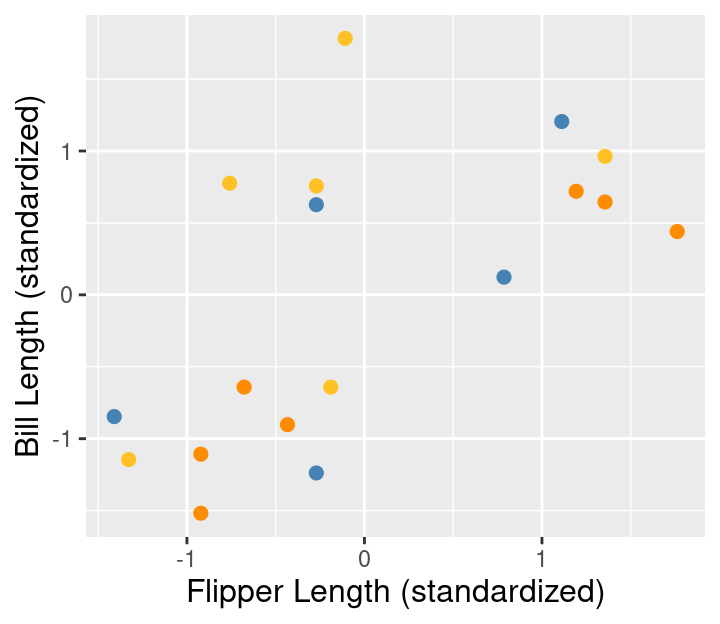Figure 9.9: Random initialization of labels.

Figure 9.10 shows what the iterations of K-means would look like with the unlucky random initialization shown in Figure 9.9.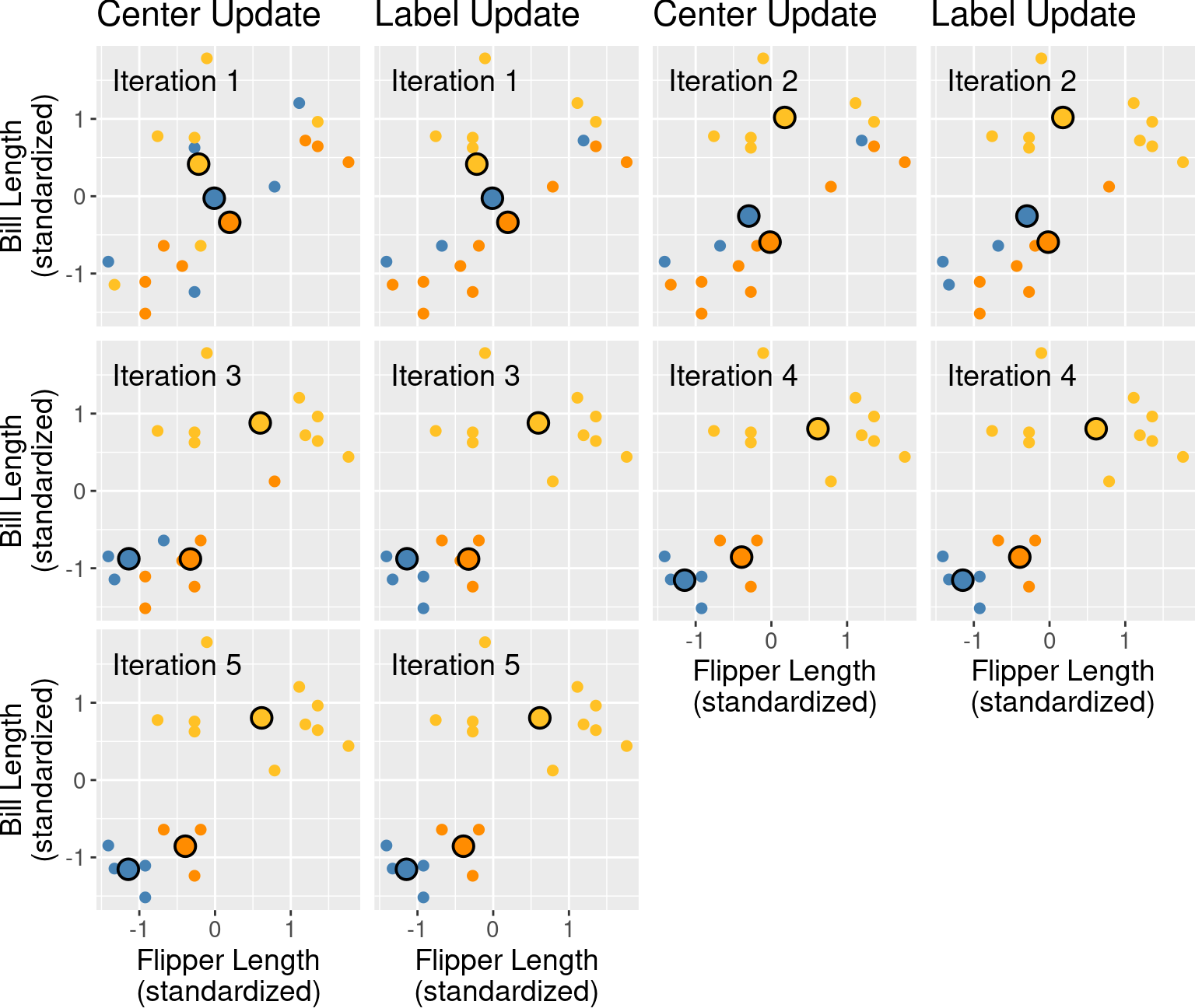Figure 9.10: First five iterations of K-means clustering on the penguin_data example data set with a poor random initialization. Each pair of plots corresponds to an iteration. Within the pair, the first plot depicts the center update, and the second plot depicts the reassignment of data to clusters. Cluster centers are indicated by larger points that are outlined in black.

This looks like a relatively bad clustering of the data, but K-means cannot improve it. To solve this problem when clustering data using K-means, we should randomly re-initialize the labels a few times, run K-means for each initialization, and pick the clustering that has the lowest final total WSSD.

### 9.4.4 Choosing K

In order to cluster data using K-means, we also have to pick the number of clusters, K. But unlike in classification, we have no response variable and cannot perform cross-validation with some measure of model prediction error. Further, if K is chosen too small, then multiple clusters get grouped together; if K is too large, then clusters get subdivided. In both cases, we will potentially miss interesting structure in the data. Figure 9.11 illustrates the impact of K on K-means clustering of our penguin flipper and bill length data by showing the different clusterings for K’s ranging from 1 to 9.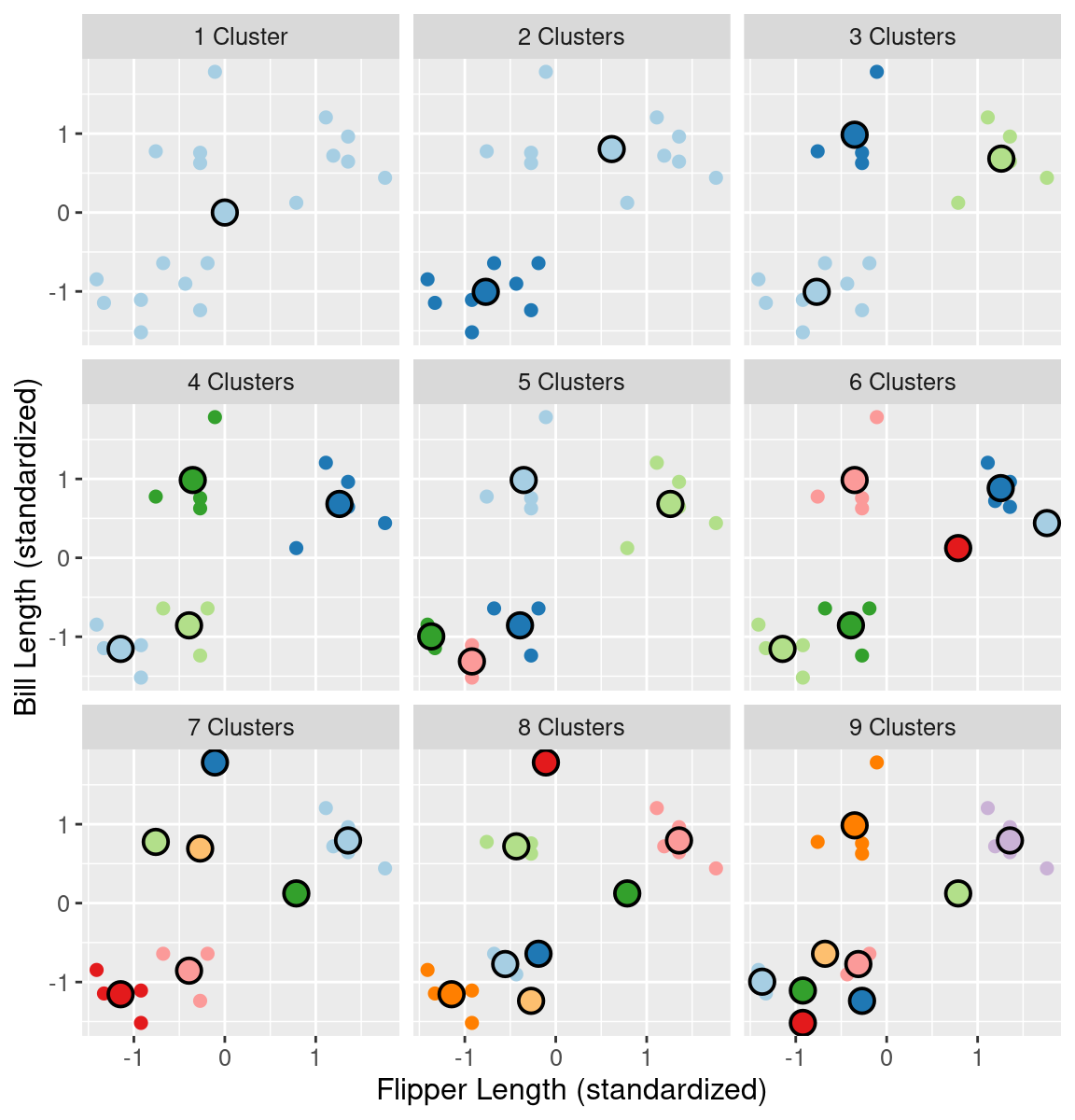Figure 9.11: Clustering of the penguin data for K clusters ranging from 1 to 9. Cluster centers are indicated by larger points that are outlined in black.

If we set K less than 3, then the clustering merges separate groups of data; this causes a large total WSSD, since the cluster center is not close to any of the data in the cluster. On the other hand, if we set K greater than 3, the clustering subdivides subgroups of data; this does indeed still decrease the total WSSD, but by only a diminishing amount. If we plot the total WSSD versus the number of clusters, we see that the decrease in total WSSD levels off (or forms an “elbow shape”) when we reach roughly the right number of clusters (Figure 9.12).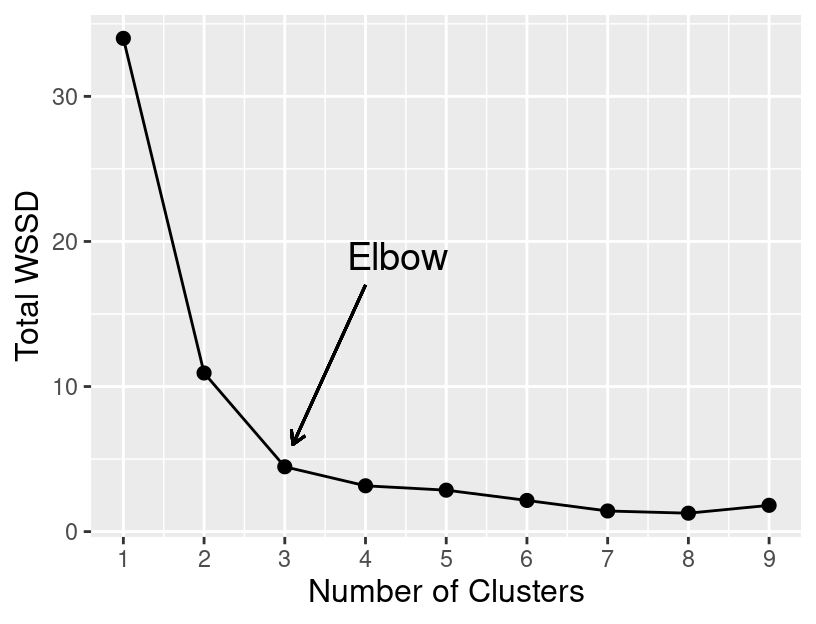Figure 9.12: Total WSSD for K clusters ranging from 1 to 9.

## 9.5 Data pre-processing for K-means

Similar to K-nearest neighbors classification and regression, K-means clustering uses straight-line distance to decide which points are similar to each other. Therefore, the scale of each of the variables in the data will influence which cluster data points end up being assigned. Variables with a large scale will have a much larger effect on deciding cluster assignment than variables with a small scale. To address this problem, we typically standardize our data before clustering, which ensures that each variable has a mean of 0 and standard deviation of 1. The scale function in R can be used to do this. We show an example of how to use this function below using an unscaled and unstandardized version of the data set in this chapter.

First, here is what the raw (i.e., not standardized) data looks like:

not_standardized_data <- read_csv("data/penguins_not_standardized.csv")
not_standardized_data
## # A tibble: 18 × 2
##    bill_length_mm flipper_length_mm
##             <dbl>             <dbl>
##  1           39.2               196
##  2           36.5               182
##  3           34.5               187
##  4           36.7               187
##  5           38.1               181
##  6           39.2               190
##  7           36                 195
##  8           37.8               193
##  9           46.5               213
## 10           46.1               215
## 11           47.8               215
## 12           45                 220
## 13           49.1               212
## 14           43.3               208
## 15           46                 195
## 16           46.7               195
## 17           52.2               197
## 18           46.8               189

And then we apply the scale function to every column in the data frame using mutate and across.

standardized_data <- not_standardized_data |>
mutate(across(everything(), scale))

standardized_data
## # A tibble: 18 × 2
##    bill_length_mm[,1] flipper_length_mm[,1]
##                 <dbl>                 <dbl>
##  1             -0.641                -0.190
##  2             -1.14                 -1.33
##  3             -1.52                 -0.922
##  4             -1.11                 -0.922
##  5             -0.847                -1.41
##  6             -0.641                -0.678
##  7             -1.24                 -0.271
##  8             -0.902                -0.434
##  9              0.720                 1.19
## 10              0.646                 1.36
## 11              0.963                 1.36
## 12              0.440                 1.76
## 13              1.21                  1.11
## 14              0.123                 0.786
## 15              0.627                -0.271
## 16              0.757                -0.271
## 17              1.78                 -0.108
## 18              0.776                -0.759

## 9.6 K-means in R

To perform K-means clustering in R, we use the kmeans function. It takes at least two arguments: the data frame containing the data you wish to cluster, and K, the number of clusters (here we choose K = 3). Note that the K-means algorithm uses a random initialization of assignments; but since we set the random seed earlier, the clustering will be reproducible.

penguin_clust <- kmeans(standardized_data, centers = 3)
penguin_clust
## K-means clustering with 3 clusters of sizes 4, 8, 6
##
## Cluster means:
##   bill_length_mm flipper_length_mm
## 1      0.9858721        -0.3524358
## 2     -1.0050404        -0.7692589
## 3      0.6828058         1.2606357
##
## Clustering vector:
##   2 2 2 2 2 2 2 2 3 3 3 3 3 3 1 1 1 1
##
## Within cluster sum of squares by cluster:
##  1.098928 2.121932 1.247042
##  (between_SS / total_SS =  86.9 %)
##
## Available components:
##
##  "cluster"      "centers"      "totss"        "withinss"     "tot.withinss"
##  "betweenss"    "size"         "iter"         "ifault"

As you can see above, the clustering object returned by kmeans has a lot of information that can be used to visualize the clusters, pick K, and evaluate the total WSSD. To obtain this information in a tidy format, we will call in help from the broom package. Let’s start by visualizing the clustering as a colored scatter plot. To do that, we use the augment function, which takes in the model and the original data frame, and returns a data frame with the data and the cluster assignments for each point:

library(broom)

clustered_data <- augment(penguin_clust, standardized_data)
clustered_data
## # A tibble: 18 × 3
##    bill_length_mm[,1] flipper_length_mm[,1] .cluster
##                 <dbl>                 <dbl> <fct>
##  1             -0.641                -0.190 2
##  2             -1.14                 -1.33  2
##  3             -1.52                 -0.922 2
##  4             -1.11                 -0.922 2
##  5             -0.847                -1.41  2
##  6             -0.641                -0.678 2
##  7             -1.24                 -0.271 2
##  8             -0.902                -0.434 2
##  9              0.720                 1.19  3
## 10              0.646                 1.36  3
## 11              0.963                 1.36  3
## 12              0.440                 1.76  3
## 13              1.21                  1.11  3
## 14              0.123                 0.786 3
## 15              0.627                -0.271 1
## 16              0.757                -0.271 1
## 17              1.78                 -0.108 1
## 18              0.776                -0.759 1

Now that we have this information in a tidy data frame, we can make a visualization of the cluster assignments for each point, as shown in Figure 9.13.

cluster_plot <- ggplot(clustered_data,
aes(x = flipper_length_mm,
y = bill_length_mm,
color = .cluster),
size = 2) +
geom_point() +
labs(x = "Flipper Length (standardized)",
y = "Bill Length (standardized)",
color = "Cluster") +
scale_color_manual(values = c("dodgerblue3",
"darkorange3",
"goldenrod1")) +
theme(text = element_text(size = 12))

cluster_plot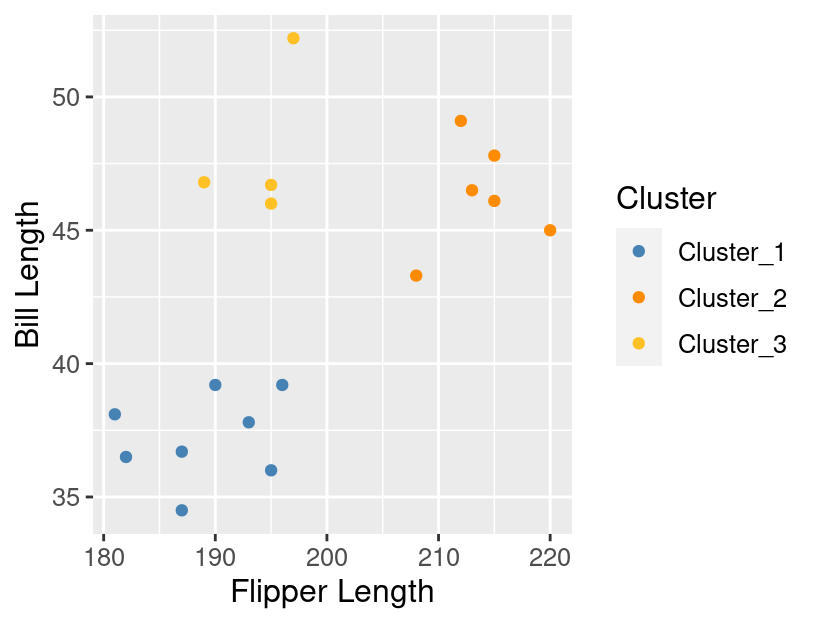Figure 9.13: The data colored by the cluster assignments returned by K-means.

As mentioned above, we also need to select K by finding where the “elbow” occurs in the plot of total WSSD versus the number of clusters. We can obtain the total WSSD (tot.withinss) from our clustering using broom’s glance function. For example:

glance(penguin_clust)
## # A tibble: 1 × 4
##   totss tot.withinss betweenss  iter
##   <dbl>        <dbl>     <dbl> <int>
## 1    34         4.47      29.5     1

To calculate the total WSSD for a variety of Ks, we will create a data frame with a column named k with rows containing each value of K we want to run K-means with (here, 1 to 9).

penguin_clust_ks <- tibble(k = 1:9)
penguin_clust_ks
## # A tibble: 9 × 1
##       k
##   <int>
## 1     1
## 2     2
## 3     3
## 4     4
## 5     5
## 6     6
## 7     7
## 8     8
## 9     9

Then we use rowwise + mutate to apply the kmeans function within each row to each K. However, given that the kmeans function returns a model object to us (not a vector), we will need to store the results as a list column. This works because both vectors and lists are legitimate data structures for data frame columns. To make this work, we have to put each model object in a list using the list function. We demonstrate how to do this below:

penguin_clust_ks <- tibble(k = 1:9) |>
rowwise() |>
mutate(penguin_clusts = list(kmeans(standardized_data, k)))

If we take a look at our data frame penguin_clust_ks now, we see that it has two columns: one with the value for K, and the other holding the clustering model object in a list column.

penguin_clust_ks
## # A tibble: 9 × 2
## # Rowwise:
##       k penguin_clusts
##   <int> <list>
## 1     1 <kmeans>
## 2     2 <kmeans>
## 3     3 <kmeans>
## 4     4 <kmeans>
## 5     5 <kmeans>
## 6     6 <kmeans>
## 7     7 <kmeans>
## 8     8 <kmeans>
## 9     9 <kmeans>

If we wanted to get one of the clusterings out of the list column in the data frame, we could use a familiar friend: pull. pull will return to us a data frame column as a simpler data structure; here, that would be a list. And then to extract the first item of the list, we can use the pluck function. We pass
it the index for the element we would like to extract (here, 1).

penguin_clust_ks |>
pull(penguin_clusts) |>
pluck(1)
## K-means clustering with 1 clusters of sizes 18
##
## Cluster means:
##   bill_length_mm flipper_length_mm
## 1   6.352943e-16     -8.203315e-16
##
## Clustering vector:
##   1 1 1 1 1 1 1 1 1 1 1 1 1 1 1 1 1 1
##
## Within cluster sum of squares by cluster:
##  34
##  (between_SS / total_SS =   0.0 %)
##
## Available components:
##
##  "cluster"      "centers"      "totss"        "withinss"     "tot.withinss"
##  "betweenss"    "size"         "iter"         "ifault"

Next, we use mutate again to apply glance to each of the K-means clustering objects to get the clustering statistics (including WSSD). The output of glance is a data frame, and so we need to create another list column (using list) for this to work. This results in a complex data frame with 3 columns, one for K, one for the K-means clustering objects, and one for the clustering statistics:

penguin_clust_ks <- tibble(k = 1:9) |>
rowwise() |>
mutate(penguin_clusts = list(kmeans(standardized_data, k)),
glanced = list(glance(penguin_clusts)))

penguin_clust_ks
## # A tibble: 9 × 3
## # Rowwise:
##       k penguin_clusts glanced
##   <int> <list>         <list>
## 1     1 <kmeans>       <tibble [1 × 4]>
## 2     2 <kmeans>       <tibble [1 × 4]>
## 3     3 <kmeans>       <tibble [1 × 4]>
## 4     4 <kmeans>       <tibble [1 × 4]>
## 5     5 <kmeans>       <tibble [1 × 4]>
## 6     6 <kmeans>       <tibble [1 × 4]>
## 7     7 <kmeans>       <tibble [1 × 4]>
## 8     8 <kmeans>       <tibble [1 × 4]>
## 9     9 <kmeans>       <tibble [1 × 4]>

Finally we extract the total WSSD from the column named glanced. Given that each item in this list column is a data frame, we will need to use the unnest function to unpack the data frames into simpler column data types.

clustering_statistics <- penguin_clust_ks |>
unnest(glanced)

clustering_statistics
## # A tibble: 9 × 6
##       k penguin_clusts totss tot.withinss betweenss  iter
##   <int> <list>         <dbl>        <dbl>     <dbl> <int>
## 1     1 <kmeans>          34       34      7.11e-15     1
## 2     2 <kmeans>          34       10.9    2.31e+ 1     1
## 3     3 <kmeans>          34        4.47   2.95e+ 1     1
## 4     4 <kmeans>          34        3.54   3.05e+ 1     1
## 5     5 <kmeans>          34        2.23   3.18e+ 1     2
## 6     6 <kmeans>          34        2.15   3.19e+ 1     3
## 7     7 <kmeans>          34        1.53   3.25e+ 1     2
## 8     8 <kmeans>          34        2.46   3.15e+ 1     1
## 9     9 <kmeans>          34        0.843  3.32e+ 1     2

Now that we have tot.withinss and k as columns in a data frame, we can make a line plot (Figure 9.14) and search for the “elbow” to find which value of K to use.

elbow_plot <- ggplot(clustering_statistics, aes(x = k, y = tot.withinss)) +
geom_point() +
geom_line() +
xlab("K") +
ylab("Total within-cluster sum of squares") +
scale_x_continuous(breaks = 1:9) +
theme(text = element_text(size = 12))

elbow_plot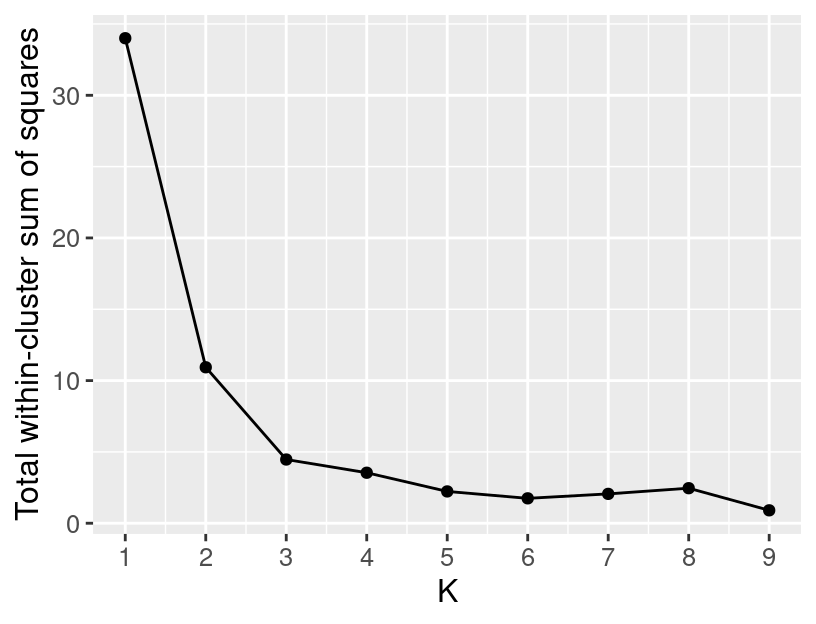Figure 9.14: A plot showing the total WSSD versus the number of clusters.

It looks like 3 clusters is the right choice for this data. But why is there a “bump” in the total WSSD plot here? Shouldn’t total WSSD always decrease as we add more clusters? Technically yes, but remember: K-means can get “stuck” in a bad solution. Unfortunately, for K = 8 we had an unlucky initialization and found a bad clustering! We can help prevent finding a bad clustering by trying a few different random initializations via the nstart argument (Figure 9.15 shows a setup where we use 10 restarts). When we do this, K-means clustering will be performed the number of times specified by the nstart argument, and R will return to us the best clustering from this. The more times we perform K-means clustering, the more likely we are to find a good clustering (if one exists). What value should you choose for nstart? The answer is that it depends on many factors: the size and characteristics of your data set, as well as how powerful your computer is. The larger the nstart value the better from an analysis perspective, but there is a trade-off that doing many clusterings could take a long time. So this is something that needs to be balanced.

penguin_clust_ks <- tibble(k = 1:9) |>
rowwise() |>
mutate(penguin_clusts = list(kmeans(standardized_data, nstart = 10, k)),
glanced = list(glance(penguin_clusts)))

clustering_statistics <- penguin_clust_ks |>
unnest(glanced)

elbow_plot <- ggplot(clustering_statistics, aes(x = k, y = tot.withinss)) +
geom_point() +
geom_line() +
xlab("K") +
ylab("Total within-cluster sum of squares") +
scale_x_continuous(breaks = 1:9) +
theme(text = element_text(size = 12))

elbow_plot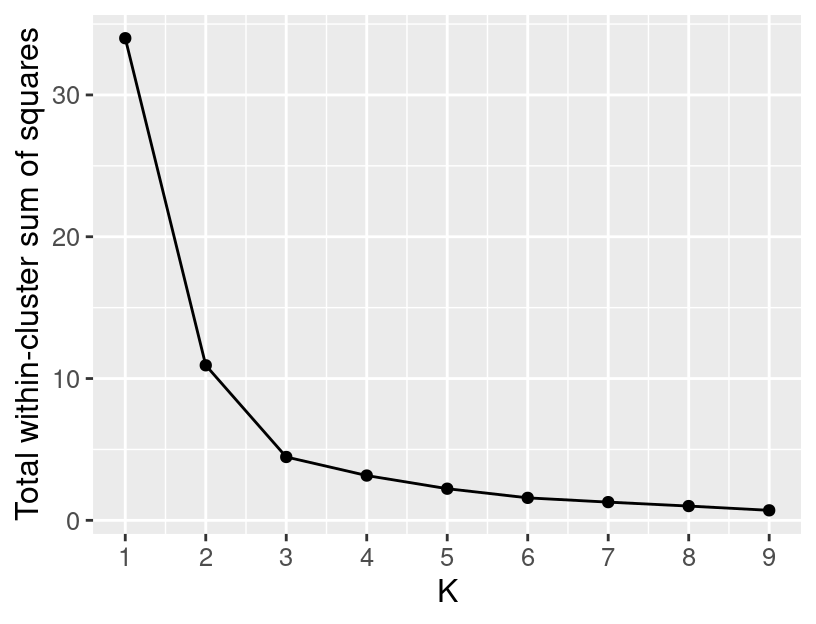Figure 9.15: A plot showing the total WSSD versus the number of clusters when K-means is run with 10 restarts.

## 9.7 Exercises

Practice exercises for the material covered in this chapter can be found in the accompanying worksheets repository in the “Clustering” row. You can launch an interactive version of the worksheet in your browser by clicking the “launch binder” button. You can also preview a non-interactive version of the worksheet by clicking “view worksheet.” If you instead decide to download the worksheet and run it on your own machine, make sure to follow the instructions for computer setup found in Chapter 13. This will ensure that the automated feedback and guidance that the worksheets provide will function as intended.

## 9.8 Additional resources

• Chapter 10 of An Introduction to Statistical Learning provides a great next stop in the process of learning about clustering and unsupervised learning in general. In the realm of clustering specifically, it provides a great companion introduction to K-means, but also covers hierarchical clustering for when you expect there to be subgroups, and then subgroups within subgroups, etc., in your data. In the realm of more general unsupervised learning, it covers principal components analysis (PCA), which is a very popular technique for reducing the number of predictors in a dataset.

### References

Gorman, Kristen, Tony Williams, and William Fraser. 2014. “Ecological Sexual Dimorphism and Environmental Variability Within a Community of Antarctic Penguins (Genus Pygoscelis).” PLoS ONE 9 (3).
Horst, Allison, Alison Hill, and Kristen Gorman. 2020. palmerpenguins: Palmer Archipelago Penguin Data. https://allisonhorst.github.io/palmerpenguins/.
James, Gareth, Daniela Witten, Trevor Hastie, and Robert Tibshirani. 2013. An Introduction to Statistical Learning. 1st ed. Springer. https://www.statlearning.com/.
Lloyd, Stuart. 1982. “Least Square Quantization in PCM.” IEEE Transactions on Information Theory 28 (2): 129–37.